SEARCH HOMEMath Central Quandaries & QueriesQuestion from James: I have the equation: tn = (n)(n+1)/2 Which gives the sequence: 1, 3, 6, 10, 15 etc. However, I'd like to be able to solve for n. So that a t of 6 would give me the answer of 3, and t of 10 would give me the answer 4 etc.We have two responses for you

James,

The first two terms differ by 2, the next two differ by 3, the next two differ by 4, etc ...
You can prove this pattern keeps going by using Pascal's identity:

For r ≥ k ≥ 0: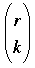=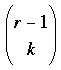+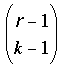, where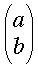= a! / (b!(a-b)!).

You can verify the identity by expanding each term using the formula above and doing
some algebra. Then you can plug in = n+1 and k = 2 to see what you get. It will be
useful to know that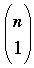= n, a fact that can also be obtained from the formula
for.

Good luck!
Victoria

Hi James. You are looking for the "inverse function" of this.

T = n(n+1)/2
2T = n² + n
0 = n² + n - 2T

Now remember the quadratic formula:
x = [-b±√(b²-4ac)] / (2a)
where a, b and c are the coefficients when x is expressed in the form 0 = ax² + bx + c.

Since we don't care about the negative roots, we just use plus instead of plus-or-minus. Let's simplify the quadratic formula using what we know from 0 = n² + n - 2T

n = [-(1)+√(²-4[2T])] / (2)
n = [-1+√(1+8T)] / 2

So there you have it. The inverse equation is
n = [-1 + √( 1 + 8T )] / 2

For example, if the total T is 15, then n is calculated:
n = [-1 + √( 1 + 8 )] / 2
n = [-1 + √( 1 + 120)] / 2
n = [-1 + √( 121 )] / 2
n = [-1 + 11] / 2
n = 10/2
n = 5, just as expected.

Cheers,
Stephen La Rocque.Math Central is supported by the University of Regina and The Pacific Institute for the Mathematical Sciences.